|

# 把纯净空气还给我们每一个家人

人离开食物可以活5个星期，离开水可以活5天，但是离开了空气却连5分钟都坚持不了，空气是我们生活中的必需品，虽然你看不到摸不着，但，它就是我们的生命。

殊不知，我们每一秒都离不开的空气，却被各种污染得我们快窒息。据世界卫生组织发出的警告，全球每年有700万人死于空气污染。与两年前的调查报告相比，此次调查显示空气污染的状况有所加剧，80%以上城市空气中颗粒物（PM10）和细颗粒物（PM2.5）污染水平超过世卫组织建议标准。2014年世卫组织公布的调查结果是基于1600多个城市提供的数据，如今已经有来自103个国家的3000多个城市提供空气监测数据。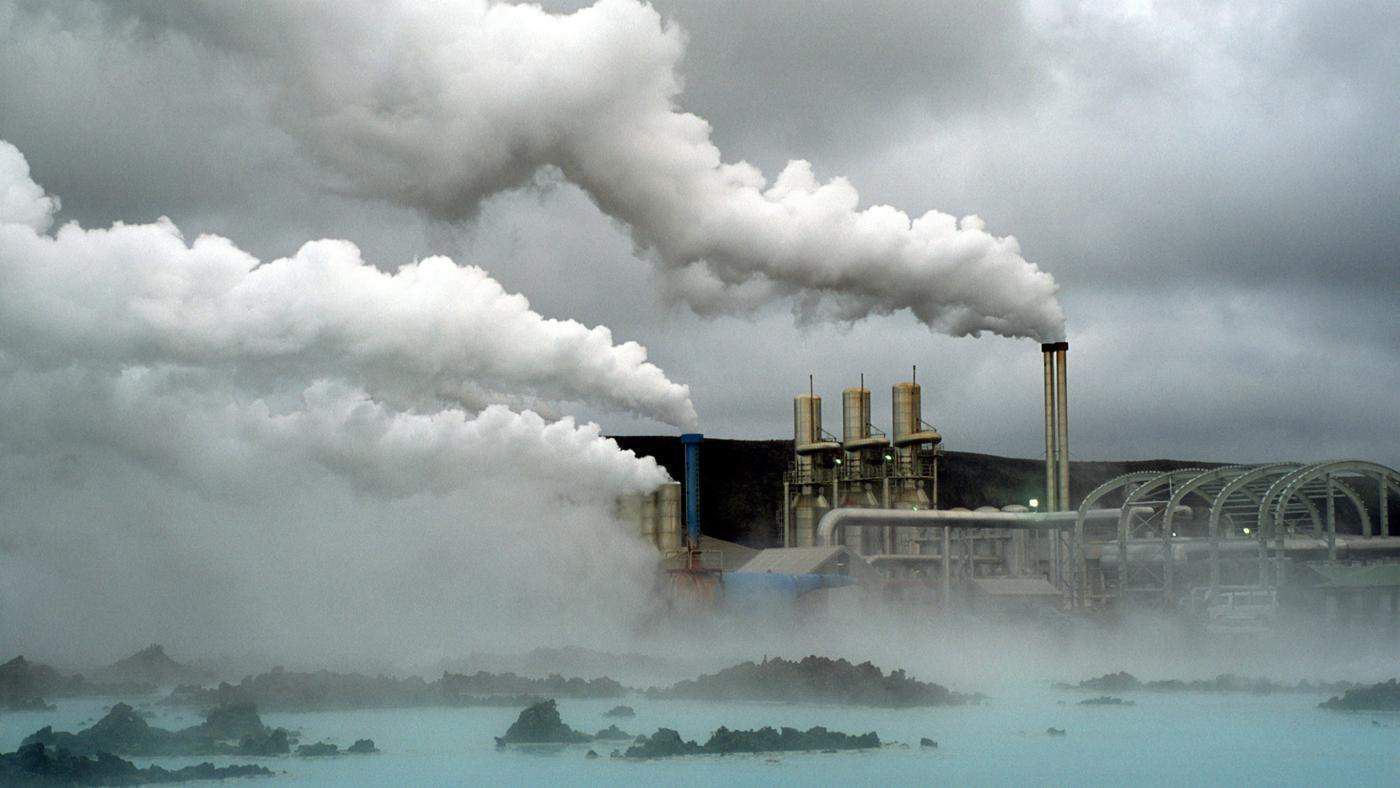大气中笼罩着浓烟滚滚的工业废气、川流不息的汽车尾气、随风飞扬的生活粉尘……室外危机，室内更需警惕。

根据英国科学家在《整体环境科学》杂志上发表论文指出，对于城市居民来说，室内空气污染所造成的健康危害远远超出了户外的空气污染。研究显示，随着建筑装修热的兴起和室内密闭程度的提高，室内空气污染物越来越多，主要包括甲醛、苯类、氡等。

1.甲醛：具有刺激性气味，尤其是人造板材、胶粘剂是甲醛污染的主要来源。甲醛释放期长达3～15年，在室内达到一定浓度时，人们就有不适感，可引起眼红、眼痒、咽喉不适或疼痛、声音嘶哑、打喷嚏、胸闷等；长期接触可引起各种慢性呼吸道疾病，引起鼻咽癌，结肠癌、新生儿染色体异常，甚至引发白血病，引起青少年记忆力和智力下降。

2.苯及甲苯、二甲苯：是一种无色、具有特殊芳香气味的液体。

苯有较高的毒性，是一种致癌物质，可导致障碍性贫血、胎儿先天性缺陷等。

3.TVOC：挥发性有机化合物(TVOC)， 主要来源于涂料、粘合剂生活垃圾等。能引起头晕、头痛、嗜睡、无力、胸闷等症状。

4.氨：极易溶于水，通常的单质形态是氡气，为无色、无味的惰性气体。通过呼吸系统进入人体后，能引起肺癌，严重时会损伤肾脏。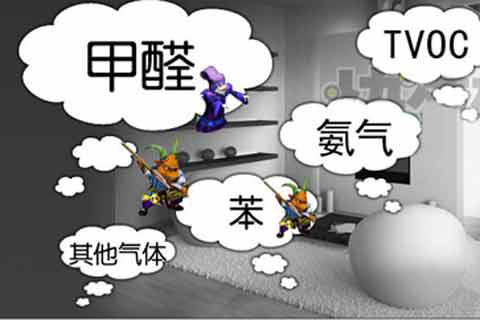室内环境污染已成为影响人类健康的一大杀手。据世界卫生组织统计，全球每年有430万例死亡事件与室内空气污染有关，因此世界卫生组织将室内空气污染列入影响人类健康的十大威胁。

2007年5月18日，陈晓旭的遗体告别仪式在深圳举行。因为乳腺癌晚期，“林妹妹”憾别人间。在铺天盖地的缅怀声中，有人惊曝陈晓旭是因家中豪华装修的大理石而患上乳腺癌。

2015年6月1日，春晚童星邓鸣贺的妹妹邓鸣璐在六一儿童节之际发长微博怀念哥哥，在微博中，她提到哥哥因为家里装修次数多才得白血病，真想揭晓，邓鸣贺患白血病的元凶竟然是油漆，一个艺术天才就这样被扼杀了。

2016年9月7日，90后女演员徐婷，因患淋巴癌离世。徐婷在弥留之际和病友说过，因为住进了刚装修完的新房，导致甲醛中毒引发淋巴癌。

一条条年轻鲜活的生命逝去，

不禁让人发问：室内污染如此可怕，是不是只能束手就擒？到底怎样才能把纯净的空气还给我们每一个家人？

一片砖，改变亿万家庭呼吸健康！

曾汉民，一个在国家高技术新材料领域如雷贯耳的名字！他对负离子材料应用问题的探索孜孜不倦，曾任中山大学校长，是国家高技术新材料领域第一届首席科学家！

2003年，特地与曾教授在进行野外考察陶瓷原矿的时候，惊喜发现某个矿场上空气特别新鲜，细究之下，他们惊喜地发现该矿场矿石具有与空气中的水反应产生负离子的功能，遂将负离子在陶瓷上的应用上达成共识，决定携手攻关，发明具有负离子功能性的瓷砖。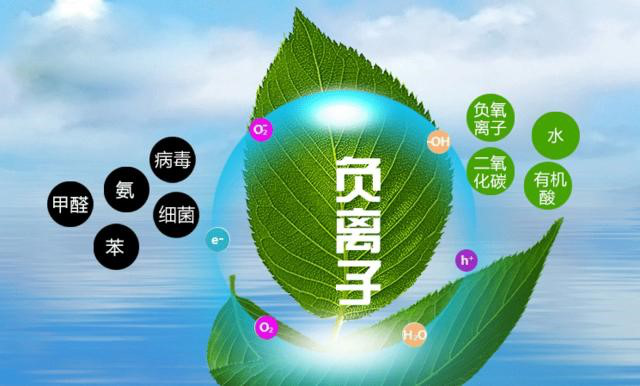但如何解决负离子材料在高温下性能稳定是曾教授与特地核心科研团队首要攻克的技术难题。研究的过程是漫长而艰难的，为此，他们进行成千上万次实验。终于，在曾教授与特地核心科研团队的努力下，2004年，他们发明了一款可产生负离子、净化空气的健康瓷砖，并于2007年行业首家获得国家发明专利授权！15年研发，12年专利，特地·负离子瓷砖为亿万家庭呼吸健康做出了革命性的改变！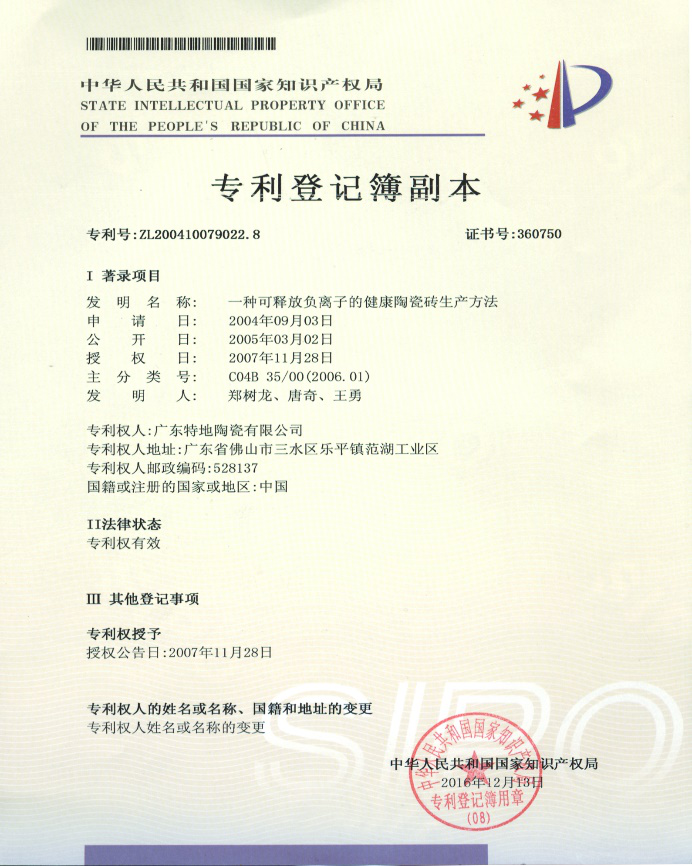什么是特地·负离子瓷砖？

特地·负离子瓷砖是一款可产生负离子、净化空气的健康瓷砖。

特地·负离子瓷砖是选用了天然矿物原料（碧玺），同时运用了国家发明专利技术，激发了空气中的水分子，从而产生大量空气负离子；负离子可有效降解空气中PM2.5、PM10、甲醛等有害物质，起到净化空气的作用。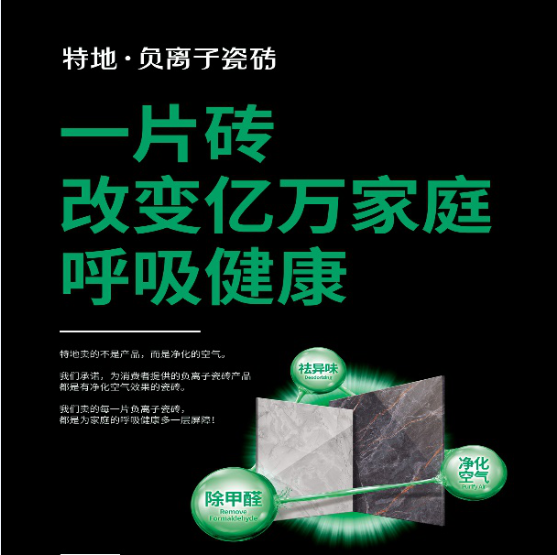负离子瓷砖有什么作用？

室内装修污染主要是粉尘、甲醛、甲苯、有害挥发气体，负离子瓷砖能够产生负离子，负离子对以上有害物质都有较好的降解作用。

负离子技术在医学、空气净化等方面已取得了显著的成果，而特地·负离子瓷砖已经拥有12年的发明专利技术，通过不断研究与检测报告显示特地·负离子瓷砖能降解空气中的PM2.5、PM10、甲醛等有害物质，起到净化空气的作用，洁净的空气可以起到改善健康的作用。

健康装修，就用特地·负离子瓷砖

我们总想给予孩子、爱人和父母最好的一切：进口玩具、名牌服饰、美味佳肴……却常常忽略了，要给他们纯净的空气，健康的居室空间！大环境的空气污染个人的力量无法改变什么，但是我们可以让自己的家做出改变，让家人的身体健康得到更好的保障。父母让我们少走弯路，我们让父母走健康之路……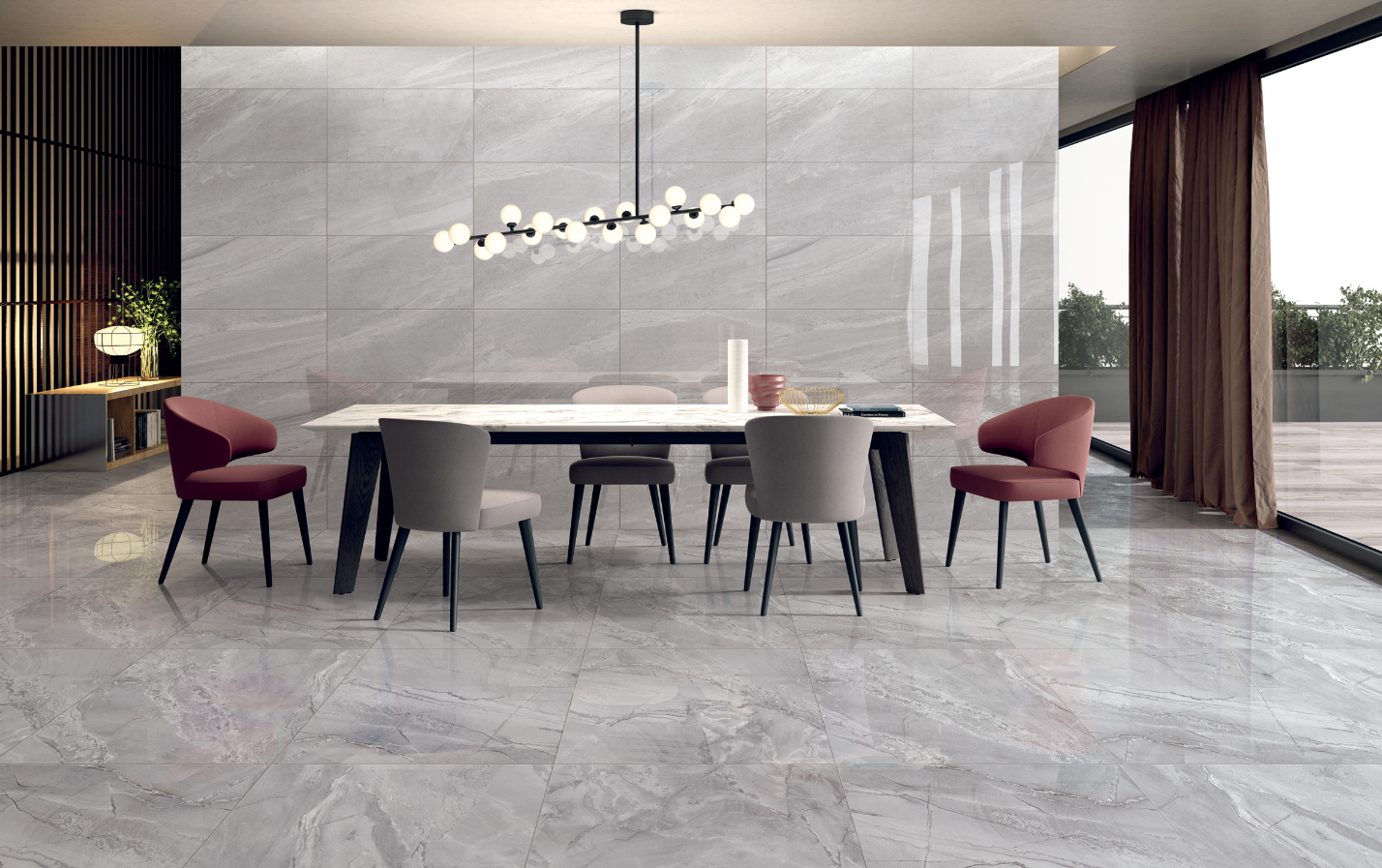`声明：本文由入驻焦点开放平台的作者撰写，除焦点官方账号外，观点仅代表作者本人，不代表焦点立场错误信息举报电话： 400-099-0099，邮箱：jubao@vip.sohu.com，或点此进行意见反馈，或点此进行举报投诉。`A B C D E F G H J K L M N P Q R S T W X Y Z
A - B - C - D - E
• A
• 鞍山
• 安庆
• 安阳
• 安顺
• 安康
• 澳门
• B
• 北京
• 保定
• 包头
• 巴彦淖尔
• 本溪
• 蚌埠
• 亳州
• 滨州
• 北海
• 百色
• 巴中
• 毕节
• 保山
• 宝鸡
• 白银
• 巴州
• C
• 承德
• 沧州
• 长治
• 赤峰
• 朝阳
• 长春
• 常州
• 滁州
• 池州
• 长沙
• 常德
• 郴州
• 潮州
• 崇左
• 重庆
• 成都
• 楚雄
• 昌都
• 慈溪
• 常熟
• D
• 大同
• 大连
• 丹东
• 大庆
• 东营
• 德州
• 东莞
• 德阳
• 达州
• 大理
• 德宏
• 定西
• 儋州
• 东平
• E
• 鄂尔多斯
• 鄂州
• 恩施
F - G - H - I - J
• F
• 抚顺
• 阜新
• 阜阳
• 福州
• 抚州
• 佛山
• 防城港
• G
• 赣州
• 广州
• 桂林
• 贵港
• 广元
• 广安
• 贵阳
• 固原
• H
• 邯郸
• 衡水
• 呼和浩特
• 呼伦贝尔
• 葫芦岛
• 哈尔滨
• 黑河
• 淮安
• 杭州
• 湖州
• 合肥
• 淮南
• 淮北
• 黄山
• 菏泽
• 鹤壁
• 黄石
• 黄冈
• 衡阳
• 怀化
• 惠州
• 河源
• 贺州
• 河池
• 海口
• 红河
• 汉中
• 海东
• 怀来
• I
• J
• 晋中
• 锦州
• 吉林
• 鸡西
• 佳木斯
• 嘉兴
• 金华
• 景德镇
• 九江
• 吉安
• 济南
• 济宁
• 焦作
• 荆门
• 荆州
• 江门
• 揭阳
• 金昌
• 酒泉
• 嘉峪关
K - L - M - N - P
• K
• 开封
• 昆明
• 昆山
• L
• 廊坊
• 临汾
• 辽阳
• 连云港
• 丽水
• 六安
• 龙岩
• 莱芜
• 临沂
• 聊城
• 洛阳
• 漯河
• 娄底
• 柳州
• 来宾
• 泸州
• 乐山
• 六盘水
• 丽江
• 临沧
• 拉萨
• 林芝
• 兰州
• 陇南
• M
• 牡丹江
• 马鞍山
• 茂名
• 梅州
• 绵阳
• 眉山
• N
• 南京
• 南通
• 宁波
• 南平
• 宁德
• 南昌
• 南阳
• 南宁
• 内江
• 南充
• P
• 盘锦
• 莆田
• 平顶山
• 濮阳
• 攀枝花
• 普洱
• 平凉
Q - R - S - T - W
• Q
• 秦皇岛
• 齐齐哈尔
• 衢州
• 泉州
• 青岛
• 清远
• 钦州
• 黔南
• 曲靖
• 庆阳
• R
• 日照
• 日喀则
• S
• 石家庄
• 沈阳
• 双鸭山
• 绥化
• 上海
• 苏州
• 宿迁
• 绍兴
• 宿州
• 三明
• 上饶
• 三门峡
• 商丘
• 十堰
• 随州
• 邵阳
• 韶关
• 深圳
• 汕头
• 汕尾
• 三亚
• 三沙
• 遂宁
• 山南
• 商洛
• 石嘴山
• T
• 天津
• 唐山
• 太原
• 通辽
• 铁岭
• 泰州
• 台州
• 铜陵
• 泰安
• 铜仁
• 铜川
• 天水
• 天门
• W
• 乌海
• 乌兰察布
• 无锡
• 温州
• 芜湖
• 潍坊
• 威海
• 武汉
• 梧州
• 渭南
• 武威
• 吴忠
• 乌鲁木齐
X - Y - Z
• X
• 邢台
• 徐州
• 宣城
• 厦门
• 新乡
• 许昌
• 信阳
• 襄阳
• 孝感
• 咸宁
• 湘潭
• 湘西
• 西双版纳
• 西安
• 咸阳
• 西宁
• 仙桃
• 西昌
• Y
• 运城
• 营口
• 盐城
• 扬州
• 鹰潭
• 宜春
• 烟台
• 宜昌
• 岳阳
• 益阳
• 永州
• 阳江
• 云浮
• 玉林
• 宜宾
• 雅安
• 玉溪
• 延安
• 榆林
• 银川
• Z
• 张家口
• 镇江
• 舟山
• 漳州
• 淄博
• 枣庄
• 郑州
• 周口
• 驻马店
• 株洲
• 张家界
• 珠海
• 湛江
• 肇庆
• 中山
• 自贡
• 资阳
• 遵义
• 昭通
• 张掖
• 中卫

1室1厅1厨1卫1阳台

1
2
3
4
5

0
1
2

1

1

0
1
2
3报名成功，资料已提交审核A B C D E F G H J K L M N P Q R S T W X Y Z
A - B - C - D - E
• A
• 鞍山
• 安庆
• 安阳
• 安顺
• 安康
• 澳门
• B
• 北京
• 保定
• 包头
• 巴彦淖尔
• 本溪
• 蚌埠
• 亳州
• 滨州
• 北海
• 百色
• 巴中
• 毕节
• 保山
• 宝鸡
• 白银
• 巴州
• C
• 承德
• 沧州
• 长治
• 赤峰
• 朝阳
• 长春
• 常州
• 滁州
• 池州
• 长沙
• 常德
• 郴州
• 潮州
• 崇左
• 重庆
• 成都
• 楚雄
• 昌都
• 慈溪
• 常熟
• D
• 大同
• 大连
• 丹东
• 大庆
• 东营
• 德州
• 东莞
• 德阳
• 达州
• 大理
• 德宏
• 定西
• 儋州
• 东平
• E
• 鄂尔多斯
• 鄂州
• 恩施
F - G - H - I - J
• F
• 抚顺
• 阜新
• 阜阳
• 福州
• 抚州
• 佛山
• 防城港
• G
• 赣州
• 广州
• 桂林
• 贵港
• 广元
• 广安
• 贵阳
• 固原
• H
• 邯郸
• 衡水
• 呼和浩特
• 呼伦贝尔
• 葫芦岛
• 哈尔滨
• 黑河
• 淮安
• 杭州
• 湖州
• 合肥
• 淮南
• 淮北
• 黄山
• 菏泽
• 鹤壁
• 黄石
• 黄冈
• 衡阳
• 怀化
• 惠州
• 河源
• 贺州
• 河池
• 海口
• 红河
• 汉中
• 海东
• 怀来
• I
• J
• 晋中
• 锦州
• 吉林
• 鸡西
• 佳木斯
• 嘉兴
• 金华
• 景德镇
• 九江
• 吉安
• 济南
• 济宁
• 焦作
• 荆门
• 荆州
• 江门
• 揭阳
• 金昌
• 酒泉
• 嘉峪关
K - L - M - N - P
• K
• 开封
• 昆明
• 昆山
• L
• 廊坊
• 临汾
• 辽阳
• 连云港
• 丽水
• 六安
• 龙岩
• 莱芜
• 临沂
• 聊城
• 洛阳
• 漯河
• 娄底
• 柳州
• 来宾
• 泸州
• 乐山
• 六盘水
• 丽江
• 临沧
• 拉萨
• 林芝
• 兰州
• 陇南
• M
• 牡丹江
• 马鞍山
• 茂名
• 梅州
• 绵阳
• 眉山
• N
• 南京
• 南通
• 宁波
• 南平
• 宁德
• 南昌
• 南阳
• 南宁
• 内江
• 南充
• P
• 盘锦
• 莆田
• 平顶山
• 濮阳
• 攀枝花
• 普洱
• 平凉
Q - R - S - T - W
• Q
• 秦皇岛
• 齐齐哈尔
• 衢州
• 泉州
• 青岛
• 清远
• 钦州
• 黔南
• 曲靖
• 庆阳
• R
• 日照
• 日喀则
• S
• 石家庄
• 沈阳
• 双鸭山
• 绥化
• 上海
• 苏州
• 宿迁
• 绍兴
• 宿州
• 三明
• 上饶
• 三门峡
• 商丘
• 十堰
• 随州
• 邵阳
• 韶关
• 深圳
• 汕头
• 汕尾
• 三亚
• 三沙
• 遂宁
• 山南
• 商洛
• 石嘴山
• T
• 天津
• 唐山
• 太原
• 通辽
• 铁岭
• 泰州
• 台州
• 铜陵
• 泰安
• 铜仁
• 铜川
• 天水
• 天门
• W
• 乌海
• 乌兰察布
• 无锡
• 温州
• 芜湖
• 潍坊
• 威海
• 武汉
• 梧州
• 渭南
• 武威
• 吴忠
• 乌鲁木齐
X - Y - Z
• X
• 邢台
• 徐州
• 宣城
• 厦门
• 新乡
• 许昌
• 信阳
• 襄阳
• 孝感
• 咸宁
• 湘潭
• 湘西
• 西双版纳
• 西安
• 咸阳
• 西宁
• 仙桃
• 西昌
• Y
• 运城
• 营口
• 盐城
• 扬州
• 鹰潭
• 宜春
• 烟台
• 宜昌
• 岳阳
• 益阳
• 永州
• 阳江
• 云浮
• 玉林
• 宜宾
• 雅安
• 玉溪
• 延安
• 榆林
• 银川
• Z
• 张家口
• 镇江
• 舟山
• 漳州
• 淄博
• 枣庄
• 郑州
• 周口
• 驻马店
• 株洲
• 张家界
• 珠海
• 湛江
• 肇庆
• 中山
• 自贡
• 资阳
• 遵义
• 昭通
• 张掖
• 中卫• 手机• 分享
• 设计
免费设计
• 计算器
装修计算器
• 入驻
合作入驻
• 联系
联系我们
• 置顶
返回顶部